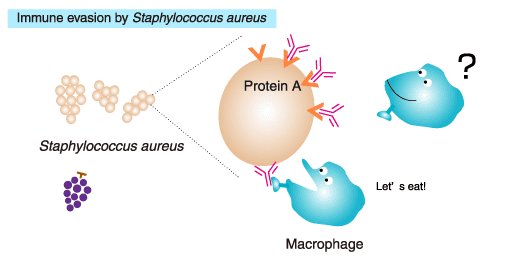## Protein A ELISA Kit

Introduction

The Protein A ELISA kit (catalogue number 9000-1) is designed for the detection and quantification of residual native and recombinant protein A (RPA). Our Protein A ELISA kit has been developed for those customers who require a highly sensitive assay to measure small amounts of contaminating protein A in antibody products. Protein A contamination testing is performed at several different stages of development and commercial manufacturing, which may include:

• Process development for resin leaching characteristics under specific conditions
• Fabrication, typically from eluted samples taken at various points in the purification process
• Complete product release to document process containment levels and batch-to-batch consistency

The following summary report contains performance data compiled from the evaluation of the Protein A ELISA Kit in the presence of human immunoglobulin G (hIgG). The data presented here demonstrate that the Protein A ELISA kit:

• Ability to detect RPA in the presence of up to 0.5 mg/mL hIgG in PBS-T buffer
• Recovery percentage (accuracy), inter-assay and intra-assay precision, the limit of quantitation and limit of detection

Summary Of Results

rPA in the presence of hIgG

The performance of the Protein A ELISA kit was evaluated by detecting RPA in the presence of hIgG compared to a standard containing no hIgG. All RPA spiked samples had a final hIgG concentration of 0.125 mg/ml (after final dilution in the assay plate). Each sample was prepared in replicates of 8 and three separate ELISAs were performed according to the kit’s standard protocol.

Limit of Quantification (LoQ)

The LoQ determined from the standard curve was calculated to be 0.037 ng/ml. The LoQ for samples spiked with RPA in the presence of hIgG was 0.102 ng/mL or 0.82 ng/mg (0.82 ppm). The sensitivity of the kit in detecting rPA50 ligand in the presence of immunoglobulin is < 1 part per million (ppm) or 0.1 ng/mL.

Conclusions

Intra-assay data indicated that the precision of the standard curve samples was within acceptable parameters with %CV values ​​ranging from 3.5 to 8.6%. The LoQ determined from the standard curve was 0.037 ng/ml.

Intra-assay data indicated that samples were analyzed with relative accuracy and precision. The calculated LoQ for the samples was 0.102 ng/mL. (The sensitivity of the Protein A ELISA kit is 0.1 ng/ml). All sample concentrations at or above this limit had %CV values ​​of 10.4 to 2.6%, below the 15% limit.

Precision, measured by average % recovery, was 93-101% for standards and 90-125% for samples.

Inter-assay data indicated that the data was reproducible. The inter-assay coefficient of variation was less than or equal to 4.9% for all standard concentrations. The samples also had a high degree of between-assay precision with %CV values ​​in the range of 5.1-0.4% for concentrations equal to and greater than the LoQ.Explanation Of Calculations

Accuracy (%CV)

Precision was calculated by determining the standard deviation between the data points of the RPA spiked sample and dividing it by the mean value. According to the text ‘Guidance for Industry: Validation of Bioanalytical Methods’, the precision should be within 15%.

Intra-assay precision

Intra-assay precision was calculated for each RPA spiked sample concentration by averaging the %CV values ​​across all assays.

Inter-assay precision

Between-assay precision was calculated for each concentration point by determining the standard deviation between the calculated results of each of the three essays and then dividing by the mean value.

Limit of Quantification (LoQ)

The limit of quantification (LoQ) was defined as 10 times the standard deviation of 0 ng/ml of sample. The standard deviation of the OD value of 0 ng/mL was multiplied by 10 and then added to the base OD value of 0 ng/mL. The LoQ was then generated by plugging the summed value into the 4-parameter fit equation of the standard curve. For each kit, LoQ was reported as ng protein A per mL (ng/mL) buffer and ng protein A per mg hIgG (ppm) for RPA-enriched samples tested in the presence of hIgG.

Detection Limit (LoD)

The limit of detection (LoD) was defined as 3 times the standard deviation of a 0 ng/ml protein A sample. The standard deviation of the OD value of 0 ng/mL was multiplied by 3 and then added to the base OD value of 0 ng/mL. The LoD was then generated by plugging the summed value into the 4-parameter fit equation of the standard curve. For each kit, an LoD was reported as ng of protein A per mL (ng/mL) of buffer and in parts per million (ppm).

Accuracy

Precision is described as the % recovery determined by the assay compared to the theoretical spiked concentration